# Writing Modbus Data with node-red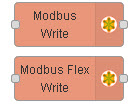In the previous tutorial we looked at the modbus nodes and looked at how to read data from a Modbus server using node-red.

In this tutorial we will look at writing data to a modus server using node-red.

### Writing Data

To write data we will use the modbus write node and modbus flex write node.

As we saw with the read nodes the write node has all of the configuration done in the node itself whereas the flex write gets the configuration from the preceding node.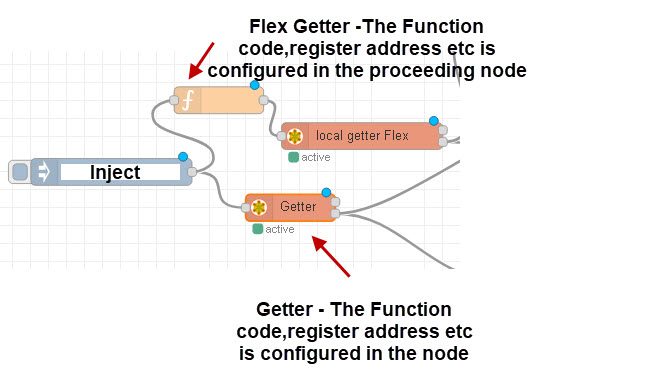The nodes support the function codes 5,6,16,16 which allow you to write to coils,registers,multiple coils and multiple registers.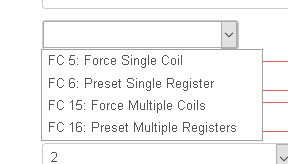When writing we have the opposite problem of reading.

### Modbus Write Examples

All of the examples use a flow like that shown below: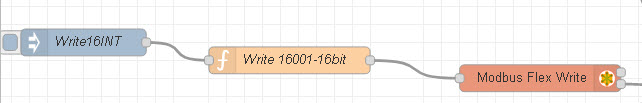The function code is used to create the write command and the code shown in the examples is placed in this node. The inject node is used just to trigger the flow but it could also contain the value to write.

### Write 16 Bit Integer

Again writing a 16 bit integer is easy as the node expects a 16 bit integer.

Below is the code from a function code that send a value of decimal 16001 to a modbus server using fc =6 (single register ) and start address of 40.

```var fc=6;
var sa=40;
var value=16001;
msg.slave_ip="192.168.1.76";
return msg;
```

You should notice that I didn’t need to manipulate buffers.

### Write 32 Bit Integer

However if we need to send a 32 bit integer then it is very different.
The code for the preceding function code is shown below were we write the value 16001 to address 50 using function code 16.The code is shown below:

```var fc=16;
var sa=50;
var buf=Buffer.alloc(4);//create buffer
buf.writeInt32BE(68001);
var values=[(buf*256+buf),(buf*256)+buf]
msg.slave_ip="192.168.1.76";
return msg;
```

This time we use fc=16 for multiple registers and we need to use 2 registers.

32 bits =4*8(bits) which is 4 buffers and so we create our empty buffer.

We then write the integer (68001) into this buffer.

For multiple resisters the node expects 16 bit decimal values for each register and so we need to split our buffer into 2 parts.

We then create an array of the form [value1,value2].

Notice value1 is the low order 2 buffers (0,1), and value 2 is the higher order buffers (2,3). Notice also we need to multiple the buffers 1 and 2 by 256.

### Write 32 Bit Float

The code for the preceding function code is shown below were we write the value 16001.5 to address 70 using function code 16.

```var fc=16;
var sa=70;
var value=16001.5;
buf=Buffer.alloc(4);
buf.writeFloatBE(value);
//buf.writeFloatBE(16001.5);
var values=[(buf*256+buf),(buf*256)+buf]
msg.slave_ip="192.168.1.31";
return msg;
```

You should notice it is almost identical to the 32bit integer.

### Write 64Bit Float

The code for the preceding function code is shown below were we write the value 16001.5 to address 80 using function code 16. Because it is 64 bits we need 8 buffers.

```var fc=16;
var sa=80;
var value=16001.5;
buf=Buffer.alloc(8);
buf.writeDoubleBE(value);
//buf.writeFloatBE(16001.5);
var values=[(buf*256+buf),(buf*256)+buf,(buf*256+buf),(buf*256)+buf];
msg.slave_ip="192.168.1.76";
return msg;
```

### Signed and Unsigned

All of the examples given above use signed integers. If you need to write signed use writeUInt16BE() and writeUInt32BE().

### BE and LE

All of the examples above use BE format. If you need LE format replace BE with LE.

I.e writeUInt16BE() becomes writeUInt16LE() etc

Video Writing data to Modbus

Resources

Flow for video2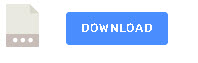Related Tutorials

Click to rate this post!
[Total: 0 Average: 0]